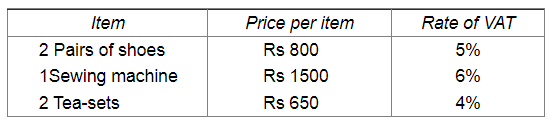# Rajat goes to a departmental store and buys the following articles:`
Question:

Rajat goes to a departmental store and buys the following articles:Calculate the total amount he has to pay to the store.

Solution:

Given,

CP of 2 pair of shoes $=$ Rs. $800 \times 2=$ Rs. 1600

Rate of VAT $=5 \%$

So, $\mathrm{VAT}=5 \%$ of Rs. $1600=\frac{5}{100} \times 1600=$ Rs. 80 .

Therefore, the amount Rajat needs to pa $y$ for 2 pair of shoes $=$ Rs. $(1600+80)=$ Rs. 1680

Again,

CP of 1 sewing machine $=$ Rs. 1500

Rate of VAT $=6 \%$

So, VAT $=6 \%$ of Rs. $1500=\frac{6}{100} \times 1500=$ Rs. 90 .

Therefore, the amount Rajat needs to pa $y$ for 1 sewing machine $=$ Rs. $(1500+90)=$ Rs. 1590

Given,

CP of 2 tea $-$ sets $=$ Rs. $650 \times 2=$ Rs. 1300

Rate of VAT $=4 \%$

So, VAT $=4 \%$ of Rs. $1300=\frac{4}{100} \times 1300=$ Rs. 52

Therefore, the amount Rajat needs to pa $y$ for 2 tea $-$ sets $=$ Rs. $(1300+52)=$ Rs. 1352

Thus, the total amount Rajat needs to pay $=$ Rs. $(1680+1590+1352)=$ Rs. 4622